# Math > Year 2

## Representing numbers up to 100

There are different ways to represent a number.
Let’s learn about different ways of representing 37.

Counters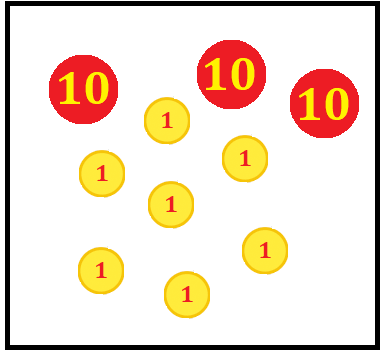Base ten Blocks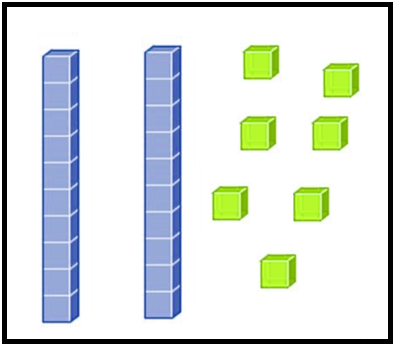Part-Whole Model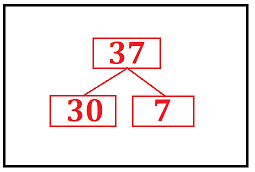Place Value Chart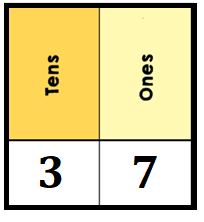Number line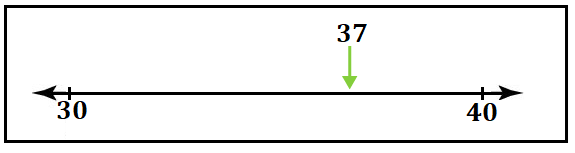Let's try some questions.

Question 1: The number represented by these counters is ______.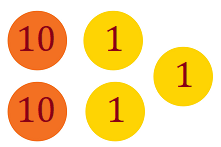Number of 10s = 2

Value of 10s = 10 + 10

= 20

Number of ones = 3

Values of ones = 1 + 1 + 1

= 3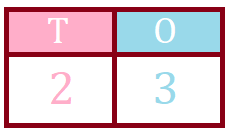Question 2: Calculate the following.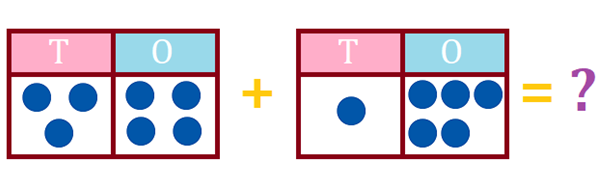The number represented by the first place value chart = 34
Tens - 3

Value of 3 tens = 10 + 10 + 10 = 30

Ones - 4

Value of 4 ones = 1 + 1 + 1 + 1  = 4

The number represented by the second place value chart = 15

Tens - 1

Value of 1 ten = 10

Ones - 5

Value of 6 ones = 1 + 1 + 1 + 1 + 1 +   = 5

Sum = 34 + 15//